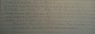# Bridge to abstract math: what is wrong with following proof

Aziza
See attached picture.

The question asks to prove that the statement which I have written on the first line is true. But I somehow proceeded to proving it is false. Basically what I did was simplify the given expression into the form (P or Q) => R and said this is equivalent to (P=>R) ^ (Q=>R). Then just looking at P=>R I arrived at a contradiction, so because F^T if F, the entire initial proposition is false. Where am I going wrong..?

#### Attachments

•mms_picture22.jpg
14.2 KB · Views: 387

UNChaneul
Interesting... $(P \lor Q) \rightarrow R$
What is the truth table for this? That is one way of showing it. Namely, what is the value of R for various values of P and Q.

Similarly, what are those values in the case of $(P \rightarrow R) \wedge (Q \rightarrow R)$

Hint: Wolfram Alpha is amazing for checking your truth tables...

Aziza
Interesting... $(P \lor Q) \rightarrow R$
What is the truth table for this? That is one way of showing it. Namely, what is the value of R for various values of P and Q.

Similarly, what are those values in the case of $(P \rightarrow R) \wedge (Q \rightarrow R)$

Hint: Wolfram Alpha is amazing for checking your truth tables...

No that's not my question...I know that this part of my proof is right. It is page 35 of my book lol. My question is that something else must be wrong with my proof

voko
Your result is correct. The statement you are trying to prove is obviously false for x = 3. I should also add that it does not seem to make much sense in the first place: it starts for "all x", but there are exactly two values of x for which it could possibly be non-trivial. Are you sure you wrote it down properly?